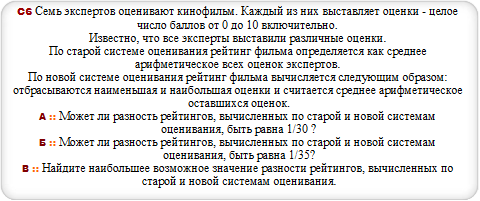# Закажи себе WolframAlpha|Pro! Получи пошаговые решения твоих задач!

## ЕГЭ с Wolfram|Alpha :: 05.06.14 :: Запад 2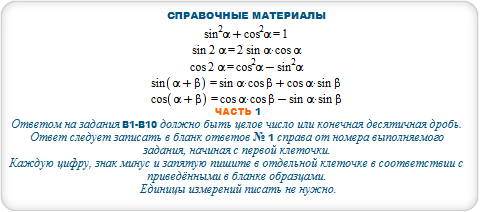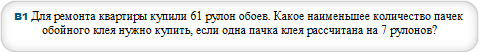#### N=ceil(z/s) where z=61,s=7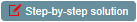#### Z=(n+mp/100)z where m=19,n=3,z=600,p=50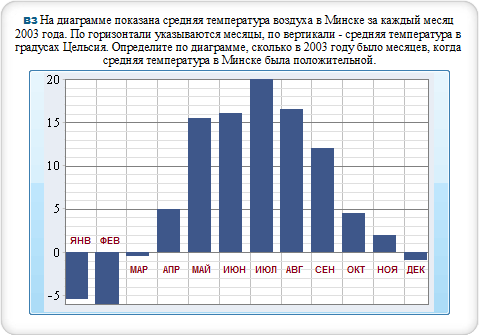#### BarChart(-5.5, -6, -0.5, 5, 15.5, 16, 20, 16.5, 12, 4.5, 2,-1)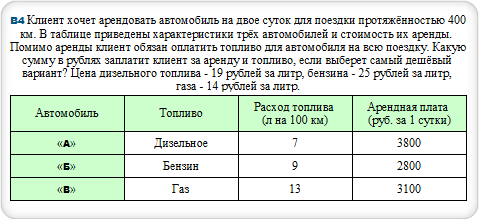#### B*n+s*v*b/100 where B=2800,b=20,v=9,s=400,n=2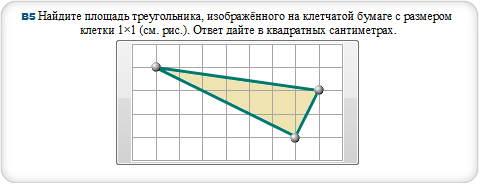#### polygon (0,0),(0,3),(7,3),(7,0), polygon (0,3),(7,2),(6,0)#### n-m where n=.98,m=.84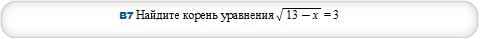#### sqrt(13-x) = 3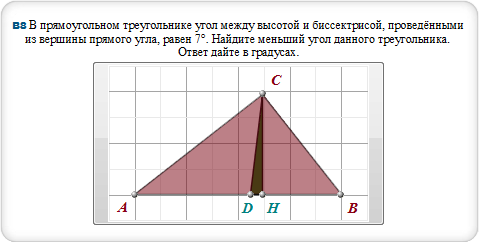#### triangle [0,0],[4,0],[2.5,1.9], triangle [2.2,0],[2.5,0.],[2.5,1.9]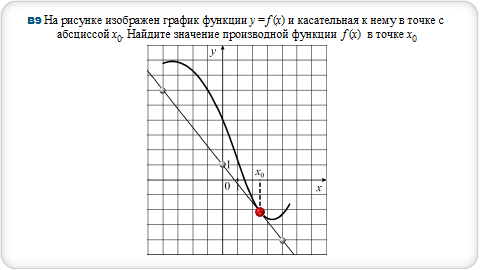#### fit polynomial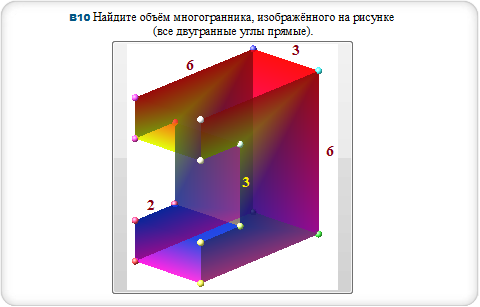#### cuboid 6,3,6, cuboid 3,3,3 and center (1.5,0,0)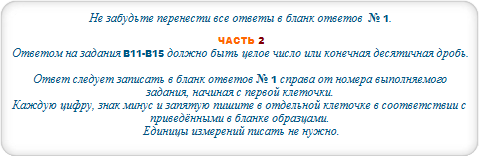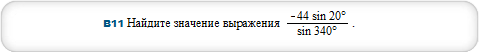#### -44 sin20/sin340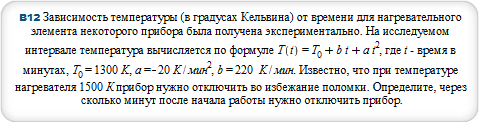#### a*t^2+b*t+Tau-T<=0 where a=-20,b=220,Tau=1300,T=1500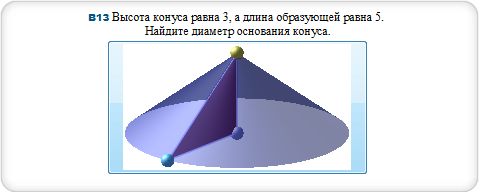#### cone with radius 3 and height 4#### {u-v = a,1/v-1/u = t/A} where A=140,a=4,t=4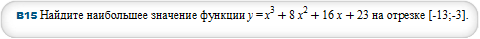#### maximize x^3+8*x^2+16*x+23 over x=-13..-3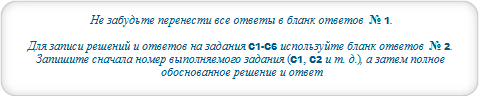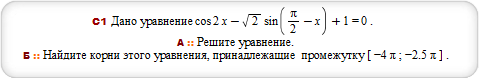#### plot (cos(2*x)+1,sqrt(2)*sin(Pi/2-x)) for x from -4*Pi to -5*Pi/2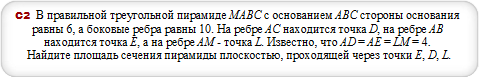#### triangular pyramid with base edge length 6, height 2*sqrt(22)#### solve {log(2-x,x+2)*log(x+3,3-x) <= 0, 4^(x^2+x-3)-(1/2)^(2*x^2-6*x-2)<=0}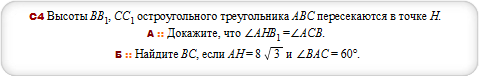#### circle (0,0),(4.1,7.1),(13.8,0) and polygon (13.8,23.9)(16.3,0),(4.1,7.1),(13.8,23.9),(13.8,0),(4.1,7.1),(0,0),(13.8,1.4)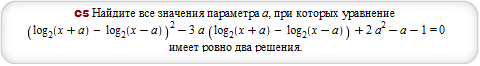#### solve (log(2,x+a)-log(2,x-a))^2-3*a*(log(2,x+a)-log(2,x-a))+2*a^2-a-1 = 0 where a=2